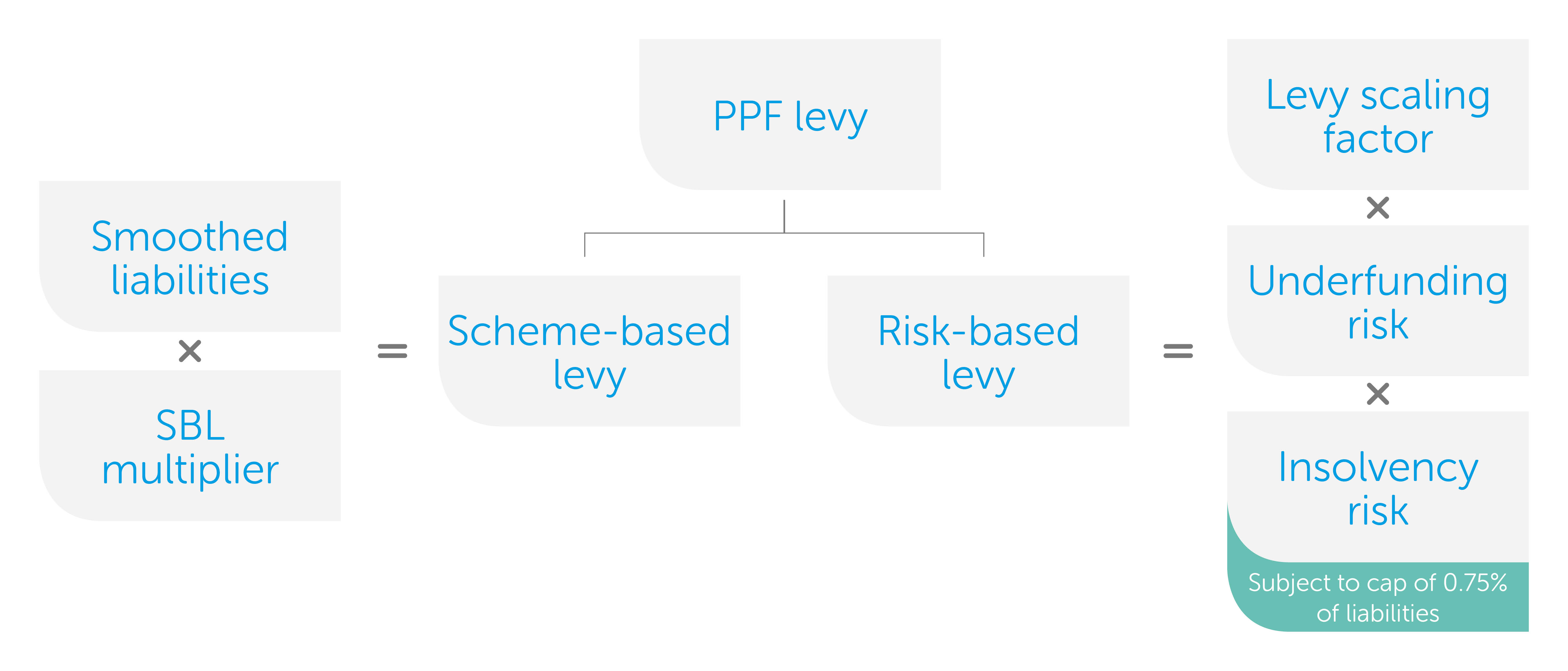The PPF levy is a function of the size of the scheme, the funding position of the scheme, and the probability of insolvency of the scheme’s sponsoring employer(s). It is calculated as follows:## Levy calculation

The PPF levy consists of two parts, which are calculated using the following formulae:

• Risk-based levy = underfunding risk x insolvency risk x levy scaling factor
• Scheme-based levy = smoothed liabilities x scheme-based multiplier

The risk-based levy is subject to a cap based on the size of the scheme’s liabilities. For the 2021/22 levy year and onwards, a “small scheme adjustment” is applied to the risk-based levy, reducing the levy for schemes with liabilities under £50 million.

## Underfunding risk

The underfunding risk is a measure of the scheme’s deficit on a basis prescribed by the PPF. It is based on the results of the most recent Section 179 valuation and the asset allocation submitted in the Scheme Return.

To ensure consistency between schemes, the asset and liability values are smoothed over a five–year period to reduce the effect of temporary market movements.

The PPF also applies a stress to the asset and liability values to illustrate how a scheme’s deficit might change under adverse market conditions. “Stressed” asset values are calculated using the asset information submitted in the annual Scheme Return. Schemes with liabilities greater than £1.5 billion are required to submit the results of a more granular asset stress calculation (referred to as a “Bespoke Stress Calculation”). This approach is also available for smaller schemes, and can help to accurately reflect the impact of liability matching investment strategies.

## Investment risk

The PPF takes a scheme’s investment strategy into account when calculating underfunding risk, to reflect the fact that some investments are more risky than others.

‘Stressed’ asset values are calculated from the asset information submitted in scheme returns. To calculate the stressed value of assets, the PPF apply a standard test to the smoothed asset value (see page 4 of the PPF’s draft investment risk appendix):

• Schemes with Section 179 liabilities that are greater than £1.5 billion are required to submit the results of some additional analysis (a 'Bespoke Stress Calculation'). This is to ensure that the investment risk for schemes that represent the largest funding risk to the PPF is reflected correctly.
• Schemes with Section 179 liabilities that are less than £1.5 billion may also carry out a bespoke stress calculation on their assets and submit the results to the PPF, but this is not compulsory. This approach tends to be most beneficial for schemes with a risk-reducing derivatives strategy in place.

## Insolvency risk

Insolvency risk is based on the average of the month-end insolvency probabilities generated by the PPF-specific insolvency risk model, which is calculated by Dun & Bradstreet (D&B). The average insolvency probability is placed into one of ten bands to reduce the sensitivity of the PPF levy to small changes in scores. The band into which the average insolvency probability is placed will determine the insolvency probability used in the levy calculation.

For schemes with more than one employer, the PPF calculate a weighted insolvency risk based on membership numbers.